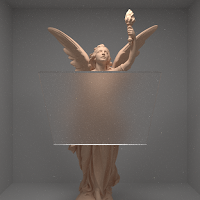### Sampling Visible Normals

The importance sampling strategy proposed in [WMLT07] gives quite acceptable results at incidence angles. However, at grazing angles some bad case scenarios may occur. For example, an incident light may reflect with high sampling weights and cause bright pixels in the output. Also, some samples are wasted due to sampling a micro normal which causes  $w_i \cdot m \lt 0$  where $w_i$ is the incident direction and $m$ is sampled micro normal. It is clear that we should also consider the incident direction $w_i$ when we are sampling micro normals $m$ for a better sampling strategy. That is, we should only sample from normals that are visible in direction $w_i$.

Importance Sampling Microfacet-Based BSDFs using the Distribution of Visible Normals[Hd14] proposed a way to sample normals from only visible normals. This strategy produces sampling weights in $[0,1]$ which is obviously better than $[0, \infty]$ of previous strategy. To better understand this paper you may refer to Understanding the Masking-Shadowing Function in Microfacet-Based BRDFs[Hei14] and these slides.

Glue uses both Walter's trick proposed in [WMLT07] and sampling of visible normals for both GGX and Beckmann distribution. It uses a boolean template parameter to decide which strategy to use in compile time.

Relevant implementations are:
The coparisons below can show the effect of sampling visible normals. If you carefully look at the glass in front of the Lucy and at neck and arms of the golden Lucy, you can see that the fireflies due to bad sampling are removed.Previous, 512spp, 370.7sec[Hd14], 512spp, 383.3secPrevious, 32spp, 19.2sec[Hd14], 32spp, 20sec